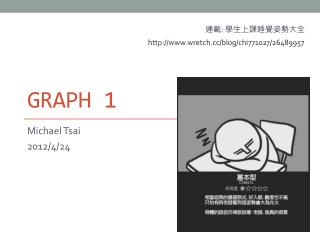DownloadDownload PresentationGraph 1

Graph 1

Download PresentationGraph 1

- - - - - - - - - - - - - - - - - - - - - - - - - - - E N D - - - - - - - - - - - - - - - - - - - - - - - - - - -
Presentation Transcript

1. 連載: 學生上課睡覺姿勢大全 http://www.wretch.cc/blog/chi771027/26489957 Graph 1 Michael Tsai 2012/4/24

2. Königsberg Seven Bridge Problem • 西元1736年, Euler嘗試著要解答這個問題: • 右邊地圖中, 有沒有可能找出一條路徑, 使得每一條橋都走過一次之後又回到出發點? 答案:Graph必須要是connected & 必須有0個或2個node有odd degree

3. A graph • G: a graph, consists of two sets, V and E. • V: a finite, nonempty set of vertices. • E: a set of pairs of vertices. • G=(V,E) • V={0,1,2,3} • E={(0,1),(0,2),(0,3),(1,2),(1,3),(2,3)} 0 2 1 3

4. Directed & undirected graph • Graph中, edge有方向的叫做directed graph, 沒方向的叫做undirected graph • Directed graph 通常又叫digraph • Undirected graph 通常就只叫做graph • (1,2) 和 (2,1) 在undirected graph是一樣的edge • <1,2>和<2,1>在digraph是不一樣的edge • V={0,1,2,3} • E=? • E={<0,1>,<0,2>,<1,2>,<2,1>,<3,1>,<3,2>} 0 2 1 3 註: 課本裡面digraph也使用() 小括號, 不過我比較喜歡用<>角括號來表示digraph的edge, 覺得這樣比較清楚.

5. Self Edge & Multigraph Graph with self-edges <v,v> Multigraph 0 0 2 2 1 1 3 3

6. Maximum number of edges • 一個有n vertices的graph, 最多有幾個edges? • 一個有n vertices的digraph, 最多有幾個edges? • 答案: graph: , • digraph:

7. 怎麼這麼多名詞XD 0 • 相鄰(adjacent): 如果有edge (u,v), 那麼u, v兩vertices就是adjacent. • 如果有edge <u,v> 那麼我們說 v is adjacent to u. (有些課本用相反的定義T_T) • 作用(incident): 如果有edge (u,v) 那麼u, v兩vertices就是incident (作用)on (在) u和 v上面 • <u,v> is incident from u and is incident to v. • <u,v> leaves u and enters v. • Subgraph: 如果G=(V,E), G’=(V’,E’)是G的subgraph, 則且 2 1 3

8. Degree of vertex • Vertex的degree: • 有幾個edge連在vertex上 • Digraph中 • 又可分為in-degree and out-degree • in-degree: 進入vertex的edge數 • out-degree: 出去vertex的edge數 • degree=in-degree+out-degree • 一個degree=0的vertex可稱為isolated • Edge數和degree的關係: 1 2 3 4 5 6

9. 路徑 (path) • Path: 一條從u到v的path, 是一連串的vertices, , 而且都是edge. (digraph的定義自行類推) • 如果有一條pathp從u到u’, 則我們說u’ is reachablefrom u via p. • Path的length: 裡面有幾條edge • Simple path: 除了u, v(起點和終點)以外, 其他的vertices都沒有重複過. • Cycle: 一個u和v一樣的simple path • Subpath:?? • 答案: 定義path的vertex sequence裡面的連續的一段. 0 2 1 3

10. 有連接的(connected) 0 半獸人 2 4 1 • In undirected graph: • Vertices u and v are said to be connectediff • there is a path from u to v. (graph & digraph) • Connected graph: • iff every pair of distinct vertices u and v in V(G) is connected • Connected component (也有人直接叫component): • A maximal connected subgraph • Maximal: no other subgraph in G is both connected and contains the component. • 問: Tree是一個怎麼樣的graph? • 答: connected and acyclic (沒有cycle) graph • 問: Forest是一個怎麼樣的graph? • 答: acyclic graph 3 5 1 2 4 5 3

11. 強連接(strongly connected) 強獸人 • In digraph: • Strongly connected: • G is strongly connected iff • for every pair of distinct vertices u and v in V • there is a directed path from u to v and also a directed path from v to u. • Strongly connected component: • A maximal subgraph which is strongly connected. 1 2 3 4 5 6

12. 要怎麼表示一個graph呢? • 主流表示法: • Adjacency matrix (用array) • Adjacency lists (用linked list) • 兩者都可以表示directed & undirected graph

13. 表示法I 用Array 1 2 3 • 本方法叫做adjacency matrix • index當作vertex號碼 • edge用matrix的值來表示: • 如果有(i,j)這條edge, 則a[i][j]=1, a[j][i]=1. (graph) • 如果有<i,j>這條edge, 則a[i][j]=1. (digraph) • 對undirected graph, (也就是對對角線對稱) • 請同學解釋要怎麼建adjacency matrix • 粉簡單. 那麼來看看好不好用: • 如果要看總共有多少條edge? O(??) • 答: • 有沒有可能跟edge數(e)成正比呢? • 通常所以adjacency matrix裡面很空 4 5 6

14. 表示法II 用linked list • 本方法叫做adjacency lists • 建立一個list arraya[n](n為vertex數目) • 每個list裡面紀錄通往別的vertex的edge • 問: 怎麼算in-degree? • 答: 如果要比較容易的話, 要另外建”inverse adjacency lists” • 請同學告訴我怎麼畫 0 1 2 3 4 5      

15. Weighted Edge 3 • Edge可以有”weight” • 表示長度, 或者是需要花費 • 一個edge有weight的graph • 又叫做network • 想想看: 用剛剛的representation要怎麼儲存weight? • 答: • adjacency matrix可以用array的值來存 • adjacency list可以在list node裡面多開一個欄位存 0 1 2 3 2 4 5 2 3 4 5 1

16. 兩者比較 • Adjacency lists: • 用來表示sparse graphs時使用比較少空間 • 使用空間: • Adjacency matrix: • 要找某個edge (u,v)有沒有在graph裡面比較快 • 一個entry只需要1 bit (unweighted graph) • 簡單容易, graph小的時候用adjacency matrix比較方便 • 使用空間:

17. Breadth-First Search (BFS) • 給一個graph G=(V,E)及一個source vertex s • 找出所有從s reachable的vertices • 計算從s到每一個reachable的vertex的最少edge數目 • 產生breadth-first tree, s為root, 而其他reachable的vertices都在樹裡面 • Directed graph & undirected graph皆可 • 此方法會先找到所有距離s distance為k的vertex, 然後再繼續找距離為k+1的vertex

18. BFS Pseudo-code v.color: 用顏色來區別discover的狀況 WHITE: 還沒discovered GRAY: discovered了, 但是和該vertex相連的鄰居還沒有都discovered BLACK: discovered了且和該vertex相連的鄰居都已discovered v.d: 和root的距離 v.pi: 祖先 (predecessor) BFS(G,s) for each vertex u.color=WHITE u.d= u.pi=NIL s.color=GRAY s.d=0 s.pi=NIL Q={} ENQUEUE(Q,s) while Q!={} u=DEQUEUE(Q) for each G.Adj[u] if v.color==WHITE v.color=GRAY v.d=u.d+1 v.pi=u ENQUEUE(Q,v) u.color=BLACK 初始所有vertex的值 初始開始search的vertex s的值 對每一個和u相連vertex

19. BFS Pseudo-code BFS(G,s) for each vertex u.color=WHITE u.d= u.pi=NIL s.color=GRAY s.d=0 s.pi=NIL Q={} ENQUEUE(Q,s) while Q!={} u=DEQUEUE(Q) for each G.Adj[u] if v.color==WHITE v.color=GRAY v.d=u.d+1 v.pi=u ENQUEUE(Q,v) u.color=BLACK

20. 證明BFS可以找到最短路徑 • 定義: s到v的最短路徑的長度(邊的數目) • Lemma 22.1: G=(V,E)是一個directed或undirected graph. s是一個任意vertex. 則對任何edge , . • 證明: • 如果從s開始, u是reachable, 那麼v也是. • 這個狀況下, sv的最短路徑只可能比短(最短路徑可能不經由u過來).不等式成立. • 如果u不是reachable的話, 那麼不等式一定成立. su最短路徑 s u v 可能有不經u的sv最短路徑

21. 證明BFS可以找到最短路徑 • Lemma 22.2: G=(V,E)是一個directed或undirected graph. 對G及vertex s跑BFS. 則結束的時候, 每一個, BFS計算的. • 證明: • 使用歸納法證明. 假設為”每一個, BFS計算的”. • 一開始把s丟進queue的時候, 成立. . 而其他的vertex .(起始條件) • 當找到由edge (u,v)找到vertex v時, 我們可以假設. (k的時候成立) • 且我們知道.(證出k+1的時候成立) • 每個v都只做以上步驟(更改v.d值)一次, 歸納法證明完成. Lemma 22.1

22. BFS用的queue 證明BFS可以找到最短路徑 u v • Lemma 22.3: 假設BFS執行在G=(V,E)上, queue裡面有以下vertices , 是queue的頭, 而是queue的尾. 則 and for . • 證明: • 使用歸納法. • 當queue一開始裡面只有s的時候成立. (起始條件) • 現在我們必須證明每次dequeue或enqueue的時候, 都還是成立. (k的時候) • (k+1的時候) • (1) dequeue的時候, 變成新的queue頭. 但. 且.其他不等式都不變. 因此成立. • (2) enqueue的時候, 新加入的vertex v變成. • 此時我們剛剛把u拿掉(當時是queue的頭). 所以應該. 所以. • 且, so . • 其他的不等式都不變, 因此成立.

23. 證明BFS可以找到最短路徑 • Corollary 22.4:vertices 和 在執行BFS時被enqueue且在之前被enqueue. 則當被enqueue的時候. • 證明: 直接從Lemma 22.3就可以得到. 因為v.d只會被指定值一次(enqueue之前).

24. 證明BFS可以找到最短路徑 • Theorem 22.5: 證明BFS正確性. BFS執行在G=(V,E)上, 從開始. BFS執行的時候會找出所有從s reachable的vertex . 結束的時候, 每個. • 證明: • (反證法)假設有一些v.d不是. 讓v是其中最小的一個. • Lemma 22.2說 , 所以現在. • 此時v一定是從s reachable, 不然. • 假設u是sv最短路徑上v的前一個vertex, 則 • (隱含意思: sv上最短路徑上su的部分也是su的最短路徑) • 因為, 所以 (已經假設v是其中最小的一個). s u sv最短路徑 v

25. 證明BFS可以找到最短路徑 • 上頁得到 • 考慮BFS從Queue裡面把u dequeue出來的時候. • u的鄰居v們, 可能是WHITE, GRAY, 或BLACK • 如果是WHITE, 則會設, 矛盾. • 如果是BLACK, 則它之前已經被dequeue過. Corollary 22.4說, 矛盾. • 如果是GRAY, 則它是剛剛dequeue某個vertex w的時候被改成GRAY的(比u dequeue的時間早). 所以. 但 Corollary 22.4說, 因此, 矛盾. • 因此原假設不成立.證明完畢.

26. 下課休息

27. 下課休息

28. Today’s Reading Assignment • Cormen B.4 (Appendix) and 22.1-22.2